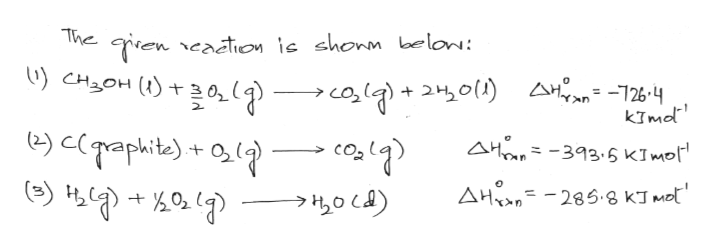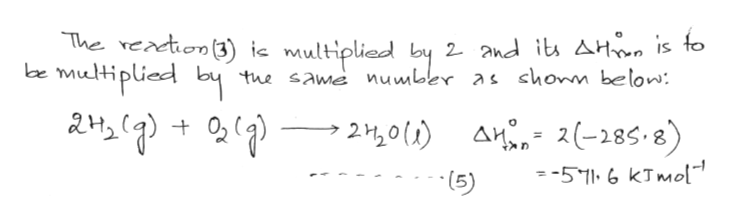# 10. From the following heats of combustion,CH3OH(l) + 3/2O2(g) → CO2(g) + 2H2O(l) ΔHorxn = –726.4 kJ/molC(graphite) + O2(g) → CO2(g) ΔHorxn = –393.5 kJ/mol H2(g) + ½O2(g) → H2O(l) ΔHorxn = –285.8 kJ/mol Calculate the enthalpy of formation of methanol (CH3OH) from its elements.C(graphite) + 2H2(g) + ½O2(g) → CH3OH(l)

Question
772 views

10. From the following heats of combustion,

CH3OH(l) + 3/2O2(g) → CO2(g) + 2H2O(l) ΔHorxn = –726.4 kJ/mol
C(graphite) + O2(g) → CO2(g) ΔHorxn = –393.5 kJ/mol
H2(g) + ½O2(g) → H2O(l) ΔHorxn = –285.8 kJ/mol

Calculate the enthalpy of formation of methanol (CH3OH) from its elements.
C(graphite) + 2H2(g) + ½O2(g) → CH3OH(l)

check_circle

Step 1help_outlineImage TranscriptioncloseTren eaction ic shonm belon: U) CHOH )20,(q) The PH+3)coig) + 2 0l) A clpraphite) 새우지 -726-4 kTmd AH 393.5 KImol 285 G KJ m AH fullscreen
Step 2
Step 3help_outlineImage TranscriptioncloseThe readtion3) ic multiplied by 2 and i AHo, is to the same number as shonn below: wultiplied by 2,0) A2(-285.8) = -571.6 kTMol (5) fullscreen

### Want to see the full answer?

See Solution

#### Want to see this answer and more?

Solutions are written by subject experts who are available 24/7. Questions are typically answered within 1 hour.*

See Solution
*Response times may vary by subject and question.
Tagged in

### Physical Chemistry